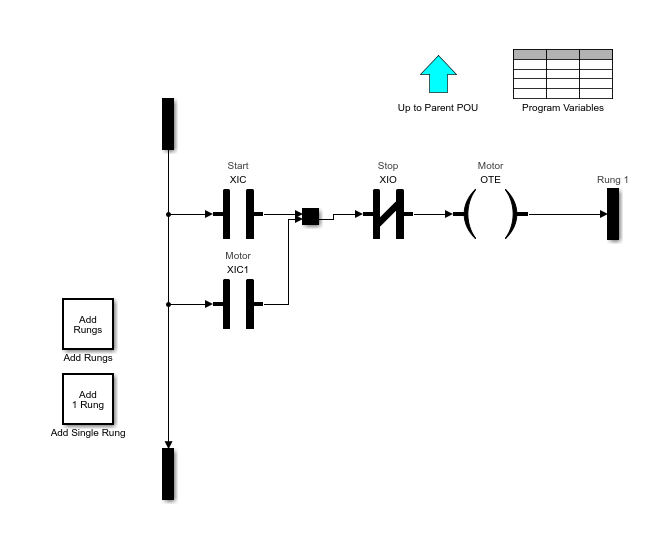The following example demonstrates how to import a simple Ladder Diagram from an `.L5X` file (`simpleController.L5X`) into the Simulink® environment and then generate Ladder Diagram (L5X) from the imported model. The Ladder Diagram `.L5X` file was created using RSLogix™ 5000 IDE and contains contacts and coils representing switches and motor. The following is a snapshot of the ladder structure.1. Use the `plcladderimport` function to import the ladder into Simulink.

`[mdlName,mdlLib,busScript] = plcimportladder('simpleController.L5X','OpenModel','On')`
2. The imported model contains a PLC Controller block named `simpleController`, followed by a Task block named `MainTask` and finally a Ladder Diagram Program block named `MainProgram`. The model imported into Simulink has blocks that implement the functionality of the contacts and coils.3. Generate code for the subsystem, `simpleController/simpleController`.

```generatedFiles = plcgeneratecode('simpleController/simpleController'); ```
```PLC code generation successful for 'simpleController/simpleController'. Generated ladder files: plcsrc\simpleController_gen.L5X```

Note

You cannot generate Structured Text code from the Ladder Diagram blocks. The Ladder feature supports only ladder code generation.